# OpenGL学习脚印: 环境纹理映射(environment mapping)

1.www.learnopengl.com cubemaps

# Reflection 反射贴图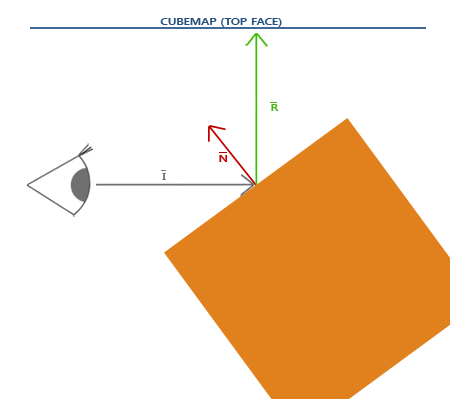#version 330 core

layout(location = 0) in vec3 position;
layout(location = 1) in vec3 normal;

uniform mat4 projection;
uniform mat4 view;
uniform mat4 model;

out vec3 FragNormal;
out vec3 FragPos;

void main()
{
gl_Position = projection * view * model * vec4(position, 1.0);
FragPos = vec3(model * vec4(position, 1.0));    // 在世界坐标系中指定
mat3 normalMatrix = mat3(transpose(inverse(model)));
FragNormal = normalMatrix * normal; // 计算法向量经过模型变换后值
}

FragPos=vec3(modelvec4(position,1.0));()$FragPos = vec3(model * vec4(position, 1.0));\tag{变换后顶点位置}$
FragNormal=mat3(transpose(inverse(model)))normal() $FragNormal = mat3(transpose(inverse(model)))*normal \tag{变换后法向量}$

   #version 330 core

in vec3 FragNormal;
in vec3 FragPos;

uniform samplerCube envText; // 环境纹理
uniform vec3 cameraPos;

out vec4 color;

void main()
{
vec3 viewDir = normalize(FragPos - cameraPos); // 注意这里向量从观察者位置指出
vec3 reflectDir = reflect(viewDir, normalize(FragNormal));
color = texture(envText, reflectDir);   // 使用反射向量采样环境纹理
}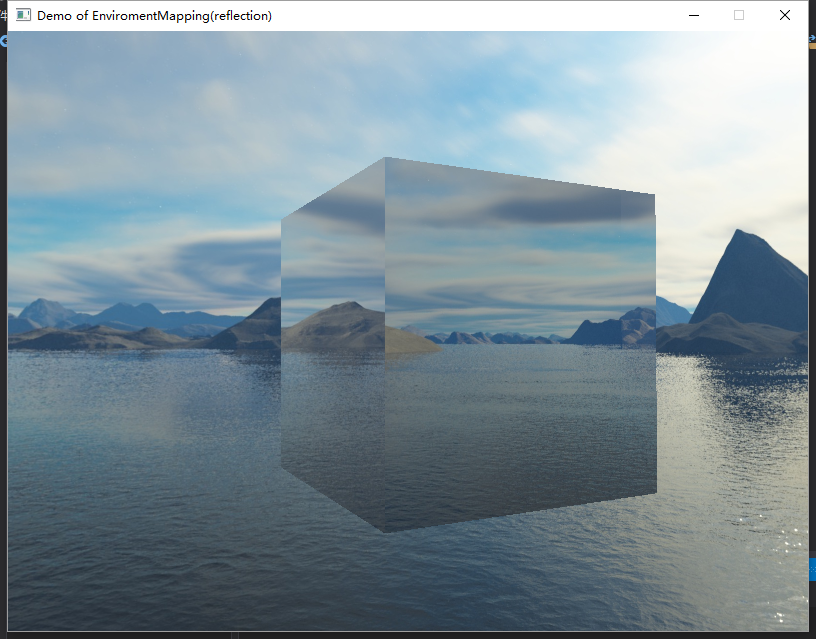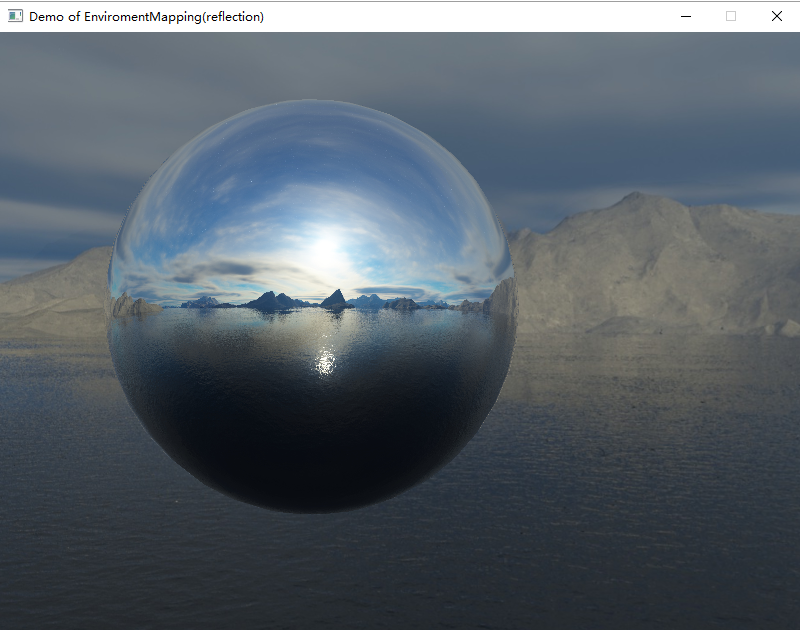# Refraction 折射贴图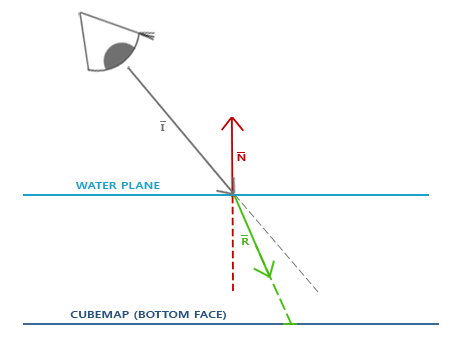1.33
1.309

   #version 330 core

in vec3 FragNormal;
in vec3 FragPos;

uniform samplerCube envText; // 环境纹理
uniform vec3 cameraPos;

out vec4 color;

void main()
{
float indexRation = 1.00 / 1.52;
vec3 viewDir = normalize(FragPos - cameraPos); // 注意这里向量从观察者位置指出
vec3 refractDir = refract(viewDir, normalize(FragNormal), indexRation);
color = texture(envText, refractDir);   // 使用 折射向量 采样环境纹理
}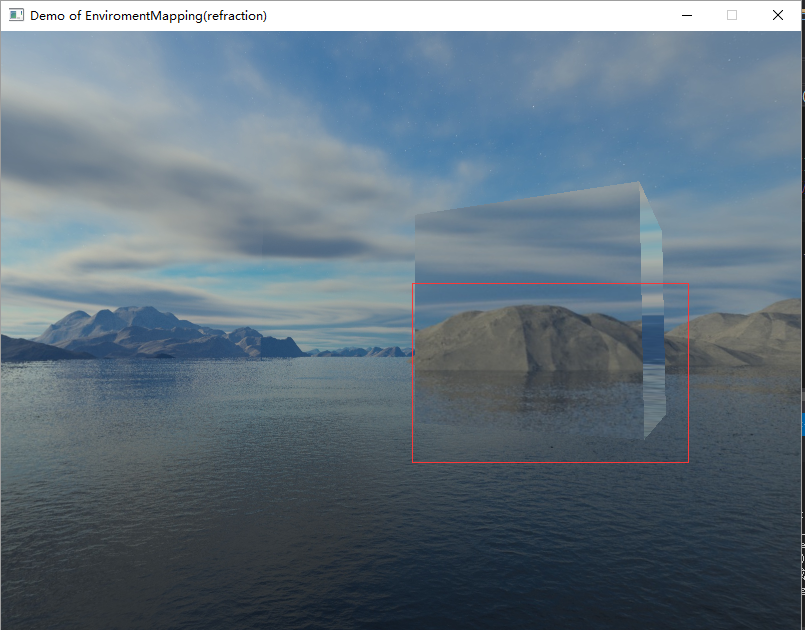# 环境纹理贴图和模型加载

// 获取Reflection 注意: AssImp对Reflection支持不好 所以这里采用ambient_map
// 除了这里的代码 还需要修改对应的obj文件
std::vector<Texture> reflectionTexture;
this->processMaterial(materialPtr, sceneObjPtr, aiTextureType_AMBIENT, reflectionTexture);
textures.insert(textures.end(), reflectionTexture.begin(), reflectionTexture.end());

#version 330 core

in vec3 FragNormal;
in vec3 FragPos;
in vec2 TextCoord;

uniform samplerCube envText;    // 环境纹理
uniform sampler2D texture_diffuse0;
uniform sampler2D specular_diffuse0;
uniform sampler2D texture_reflection0; // 反射map
uniform vec3 cameraPos;

out vec4 color;

void main()
{
vec4    diffuseColor = texture(texture_diffuse0, TextCoord);
float   relefctIntensity = texture(texture_reflection0, TextCoord).r;
vec4    reflectColor = vec4(0.0, 0.0, 0.0, 0.0);
if(relefctIntensity > 0.1) // 决定是否开启
{
vec3 viewDir = normalize(FragPos - cameraPos);
vec3 reflectDir = reflect(viewDir, normalize(FragNormal));
reflectColor = texture(envText, reflectDir) * relefctIntensity; // 使用反射向量采样环境纹理 使用强度系数控制
}
color = diffuseColor + reflectColor;
}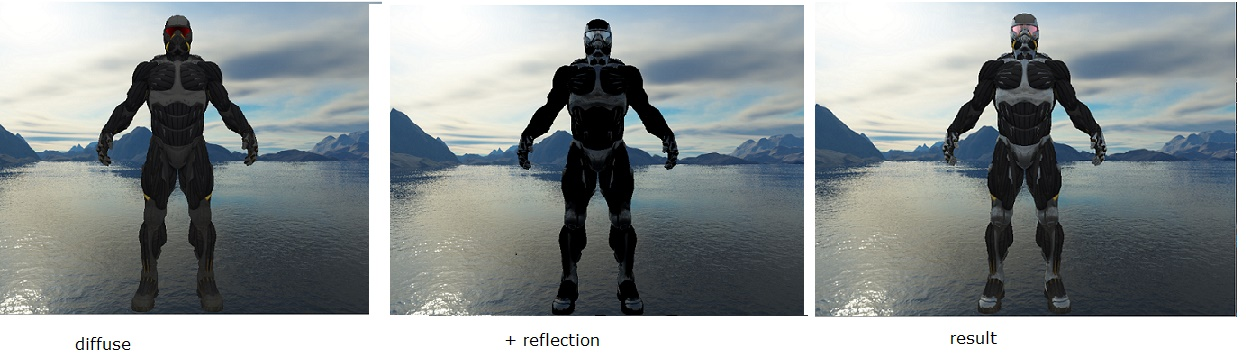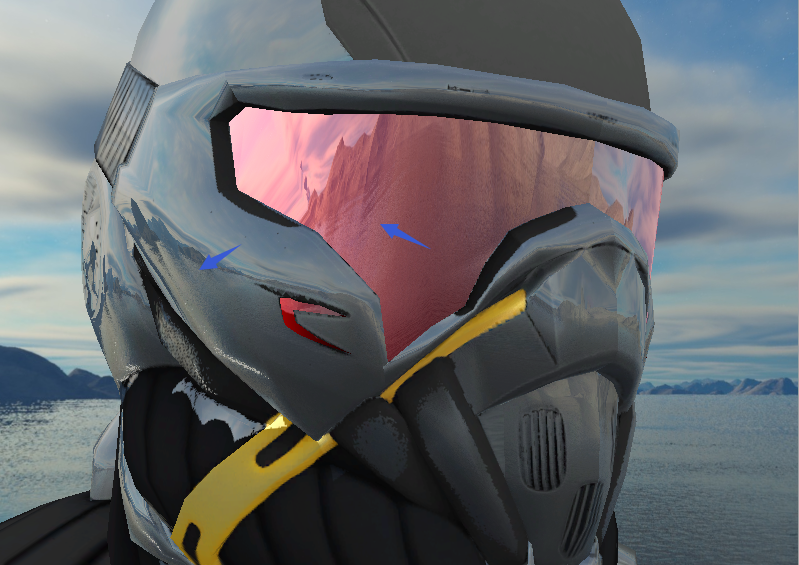# 最后的说明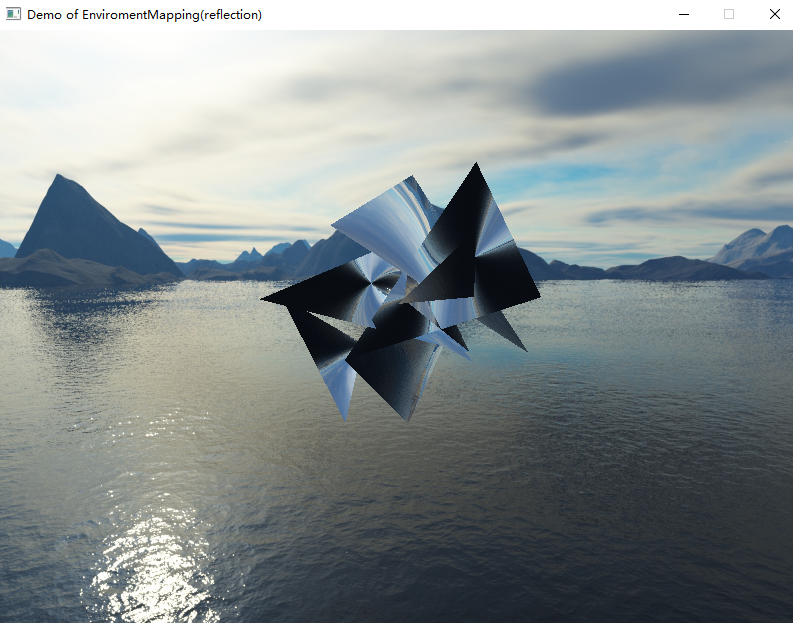glActiveTexture(GL_TEXTURE3);
glBindTexture(GL_TEXTURE_CUBE_MAP, skybox.getTextId());
glUniform1i(glGetUniformLocation(shader.programId, "envText"), 3);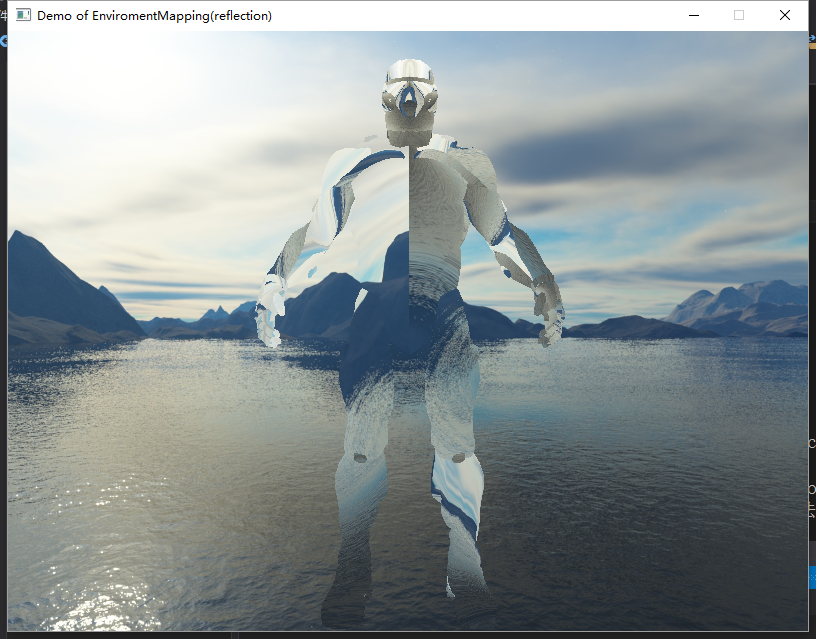01-2070405-17
03-281542
04-20104
11-301299
05-089661
04-245318
01-062686
08-04852